Visitors Online: 77 | Wednesday 18th September 2019

CBSE Guess > Papers > Question Papers > Class XII > 2003 > Physics > Compartment Outside Delhi Set -I

PHYSICS—2003 (Set I—Compartment Outside Delhi)

Q. 1. Two stars X and Y with magnitudes m1 and m2 have values of their brightness t 1 and t 2 respectively. Write expression for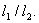Q. 2. State the reason why soft iron is used in making electromagnets.

Q. 3. Two conductors, one having resistance R and another 2R, are connected in turn across a d.c. source. If the rate of heat produced in the two conductors is Q1 and Q2 respectively, what is the value of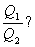Q. 4. What should be the length of a dipole antenna for a carrier wave having frequency 3 x 108 Hz?

Q. 5. How docs the intensity 'I' of scattered light vary with the wavelength of the incident light?

Q. 6. What is a button cell? Mention its two applications.

Q. 7. Derive an expression for the mutual inductance of a pair of coaxial solenoids having number of turns N1 and N2 .

Q. 8. Briefly explain, how the drift velocity of electrons in a metallic conductor varies, when (i) the temperature of the conductor is increased, and (ii) applied potential difference is decreased, keeping temperature constant.

Q. 9. A radioactive nucleus *A' decays as given below: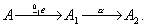If the mass number and atomic number of A 1 are 180 and 73 respectively, find the mass number and atomic number of A and A2 .

Q. 10. The intensity of solar radiation on the earth's surface is 1.4 x 103 Wm -2 . Calculate the surface temperature of earth, assuming that it re-emits all the radiations it receives from the sun.

Q. 11. State Gauss' theorem in electrostatics. A charge of 17.7 x 10 -4 C is distributed uniformly over a large sheet of area 200 m2 . Calculate the electric field intensity at a distance 20 cm from it in air.

Or

Electric field intensity at point 'B' due to a point charge 'Q' kept at point 'A' is 24 NC-1 and the electric potential at point 'B' due to same charge is 12 JC-1 . Calculate the distance AB and also the magnitude of charge Q.

Q. 12. Name the 2-input logic gate, whose truth table is given below:

 A B Output 0 0 1 1 0 1 0 1 1 1 0 0

If this logic gate is connected to a NOT gate, what will be the output when (i) A: 1, B = 1 and (ii) A = 0, B = 1?

Q. 13. Three light rays red (R), green (G) and blue (B) are incident on a right angled prism 'abc', at face 'ab'. The refractive indices of the material of the prism for red, green and blue wavelengths are 1.39, 1.44 and 1.47 respectively. Out of the three, which colour ray will emerge out of face 'ac'? Justify your answer. Trace the path of green (G) ray after passing through face 'ab'.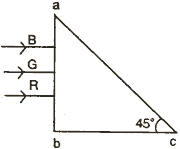Q. 14. State the postulates of Huygens' wave theory. Sketch the wave front that corresponds to a beam of light (i) coming from a very faraway source, and (ii) diverging radially from a point source.

Q. 15. Find the equivalent capacitance between the points A and B of the given combination of capacitors.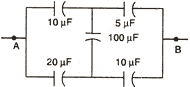If a battery of e.m.f. 10 V is connected between the points A and B, calculate the total charge in the circuit.

Q. 16. Show graphically the intensity distribution of a single slit diffraction pattern.
State with reason, how would the linear width of central maximum change if (i) monochromatic yellow light is replaced with red light, and (ii) distance between the slit and the screen is increased.

Q. 17. A metallic rod of length 'l' and resistance 'R' is moving normal to a uniform magnetic field 'B' with a velocity V. Deduce expressions for (i) the e.m.f. induced, and (ii) the induced current, in the metallic rod.

Q. 18. Figure shows how the reactance of an inductor varies with frequency.
(i) Calculate the value of the inductance of the inductor using the information given in the graph.
(ii) If this inductor is connected in series to a resistor of 8 ohm, find what would be the impedance at 300 Hz.Q. 19. An electric dipole is held in a uniform electric field.
(i) Show that no translatory force acts on it. 1
(ii) Derive an expression for the torque acting on it. 2
(iii) The dipole is aligned parallel to the field. Calculate the work done in rotating it through 1800.
Or
(i) Derive an expression for the energy stored in a parallel plate capacitor with air as the medium between its plates. 3
(ii) Air is replaced by a dielectric medium of dielectric constant 'k'. How does it change the total energy of the capacitor? 2

Q. 20. A convex lens made of material of refractive index 'n 2 ' is held in a reference medium of refractive index 'n1 '. Trace the path of a parallel beam of light passing through the lens when (i) n 1 = n2 , (ii) n1 < n2 and (iii) n1 > n2 .

Q. 21. A long straight conductor 'PQ', carrying a current of 75 A, is fixed horizontally. Another long conductor 'XV is kept parallel to 'PQ' at a distance of 5 mm, in air. Conductor 'XY' is free to move and carries a current 'I'. Calculate the magnitude and direction of current 'I' for which the magnetic repulsion just balances the weight of conductor 'XY'. (Mass per unit length for conductor 'XY' is 10 -2 kg/m)An infinitely long straight conductor 'XY' is carrying a current of 5 A. An electron is moving with a speed of 10s m/s parallel to the conductor in air from point A to B, as shown in figure. The perpendicular distance between the electron and the conductor 'XY' is 20 cm. Calculate the magnitude of the force experienced by the electron. Write the direction of this force.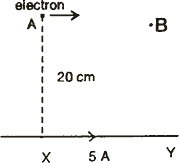Q. 22. A diamagnetic material and a paramagnetic material of the same shape and size are in turn kept in an external uniform magnetic field. Draw the modification of the magnetic lines of force in the two cases.
How does the intensity of magnetisation of a paramagnetic material vary with temperature?

Q. 23. Mention the frequency at which T.V. signals are transmitted.
Derive an expression for the range upto which signals transmitted by a T.V. tower can be received.

Q. 24. Briefly explain the following terms:
(i) Pulse-amplitude modulation (PAM), and
(ii) Pulse-code modulation (PCM).
Give one reason, why pulse-code modulation (PCM) is preferred in transmitting signals.

Q. 25. Red light, however bright, cannot cause emission of electrons from a clean zinc surface. But even weak ultraviolet radiations can do so. Why?
Draw the variation of maximum kinetic energy of emitted electrons with the frequency of incident radiation on a photosensitive surface. On the graph down, what do the following indicate (i) slope of the graph and (ii) intercept on energy axis?

Q. 26. Draw a labelled circuit diagram of common-emitter amplifier using a n-p-n transistor.
Write the expression for its voltage gain. Explain why the input and the output signals arc out of phase.

Q. 27. State Kirchhoff's laws for an electrical network.
Using Kirchhoff's laws, find the relation between the resistances of four arms of a Wheatstone bridge when the bridge is balanced.
Draw a circuit diagram to determine the unknown resistance of a metallic conductor using a metre bridge.

Or

The variation of potential difference with length in case of two potentiometers A and B is shown in the figure. Which of the two is preferred to find e.m.f. of a cell? Give reason for your answer.With the help of a circuit diagram, describe how e.m.f.'s of two primary cells can be compared by using a potentiometer.
Write two possible causes of potentiometer giving only one sided deflection in this method.

Physics 2003 Question Papers Class XII

CBSE 2003 Question Papers Class XII